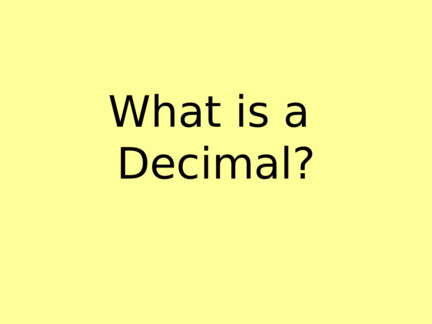# Introduction to DecimalsContributed by:In this pdf we will discuss about decimals. A decimal may have both a whole-number part and a fractional part. The whole-number part of a decimal are those digits to the left of the decimal point. The fractional part of a decimal is represented by the digits to the right of the decimal point.
1. What is a
2. What is a decimal?
A decimal is similar to a fraction –
It’s not a whole number.
Rather than saying ½, we can say 0.50
Instead of writing 1/10, we can say
one-tenth or 0.1.
It’s simply another form of a fraction.
3. What is a decimal?
A decimal is a part of a number. We use
decimals most often when we are talking
\$13.45
How would you read this number?
4. \$13.45
Thirteen dollars and forty five cents.
Remember:
You do not need to use the ¢ when your
money amount is greater than \$1.
5. What does the .45 mean?
.45 is the same thing as saying 45¢ .
It means that it is only a part of a dollar.
It is not the whole dollar.
6. Why do I have to understand
decimals?
This is a good question.
We need to understand what the value of
each number means in order to
understand what we are talking about.
Here is an example:
A shirt costs \$12.05, but when I wrote the
number down I wrote \$12.5.
What’s wrong with this?
7. Why is \$12.5 wrong?
\$12.5 really is saying \$12.50, because when we
read how much something costs it always has
two places after the decimal point.
If we don’t have a digit after the first number we
must assume it is a zero. However, when we
read \$12.05, the zero is the place holder so we
know it is 5¢ and not 50¢. That is a 45¢
difference. I can get a piece of gum for that
amount!
8. Let’s Look at Some Place Value
One Ten One Ten Cents
Hundred Dollars Dollar Cents
1 3 7 8 2
Hundreds Tens Ones Tenths Hundredths
1 3 7 8 2
AND
9. How do I read a decimal?
If you look at the number 12.3, you say:
Twelve and three tenths.
If you look at the number 12.35, you say:
Twelve and thirty-five hundredths.
If you look at a number 12.05, you say:
Twelve and five hundredths.
Now you practice saying them!
10. Let’s Write these Numbers in
Expanded Form
If we are using money, it would look
like this:
\$100 + \$30 + \$7 + 80¢ + 2¢ = \$137.82
If we are using decimals, it would
look like this:
100 + 30 + 7 + .8 + .02 = 137.82
11. How do I Compare and Order
decimals?
7.3 and 7.03 – Which is larger?
ORGANIZE – Write the numbers vertically by lining up the decimal
points.
7.3
7.03
EQUALIZE – Add a zero to make the numbers all have the same
number of digits
7.30
7.03
7.30 > 7.03
12. Compare and Order –
Another Example
Order from least to greatest: 12.34; 12.4; 12.43; 12.3
ORGANIZE – Write the numbers vertically by lining up the decimal points.
12.34
12.4
12.43
12.3
EQUALIZE – Add a zero to make the numbers all have the same number of digits
12.34
12.40
12.43
12.30
ORDER – Start with the largest place and write the decimals in order.
12.30; 12.34; 12.40; 12.43
13. When else do we use decimals?
• Weight (He weighed 85.5 lbs)
• Temperature (It was so cold today, it was
only 43.7°)
• Measuring distances (example: the race
was a 5 kilometers or 3.1 miles long
• Can you think of any other times?